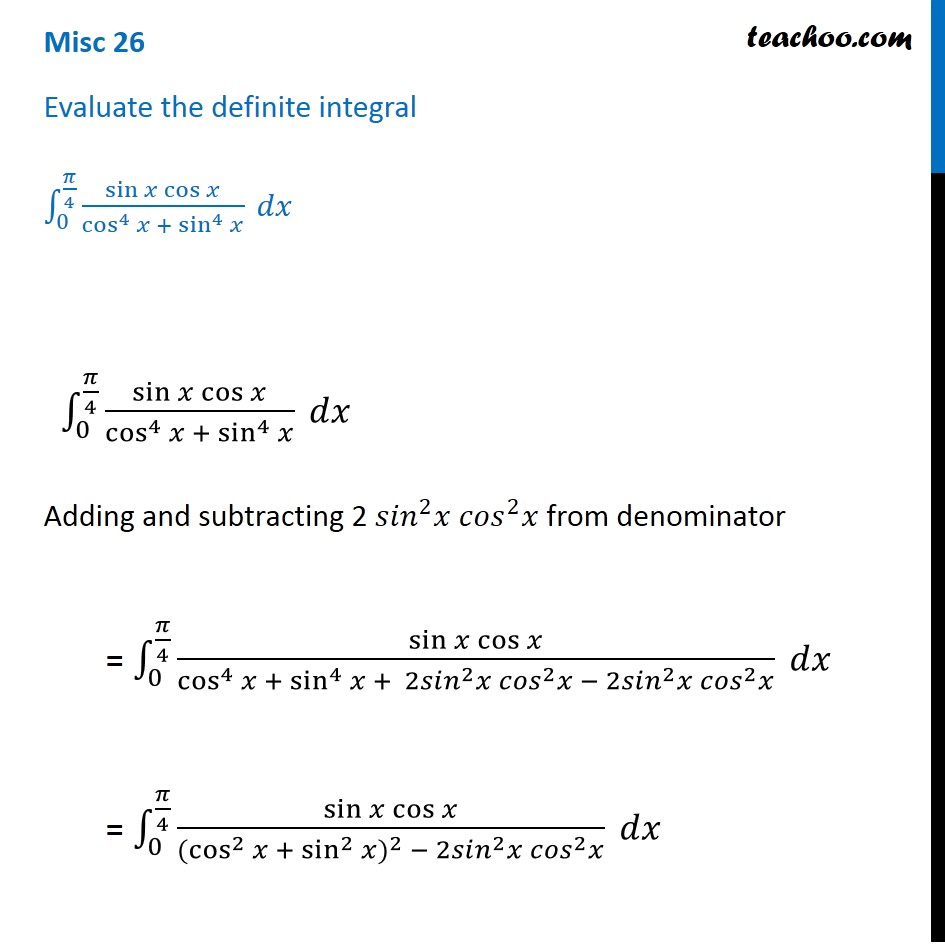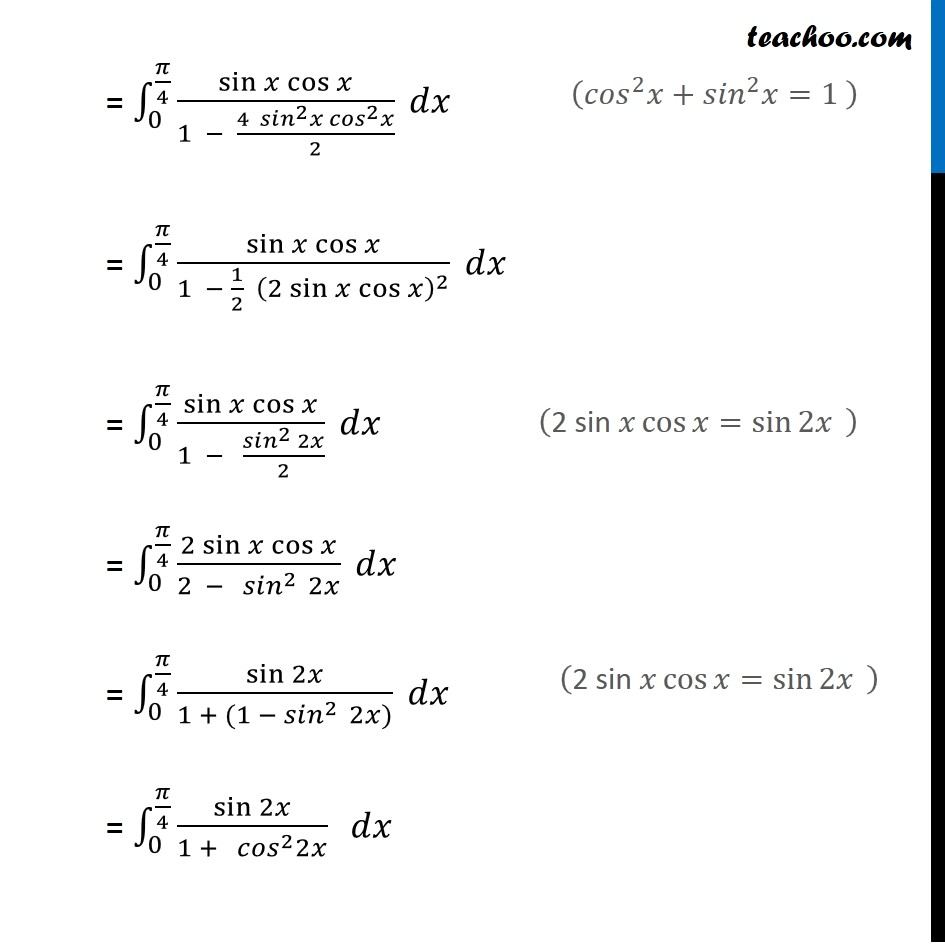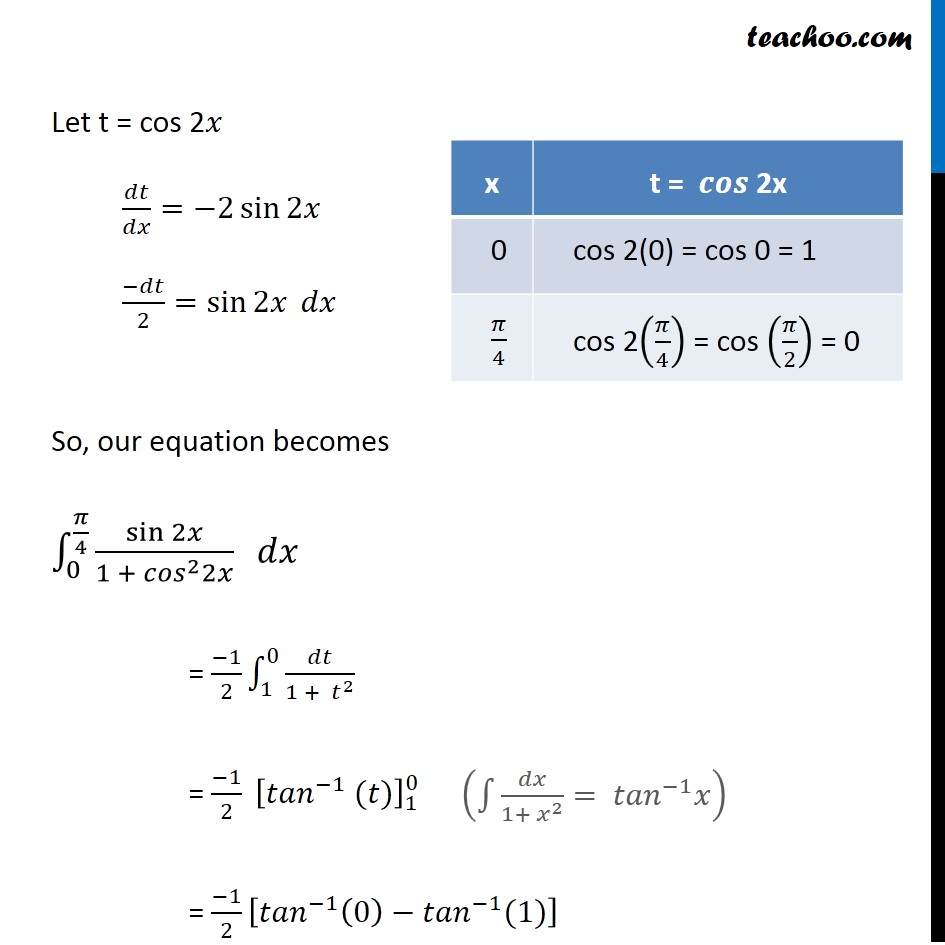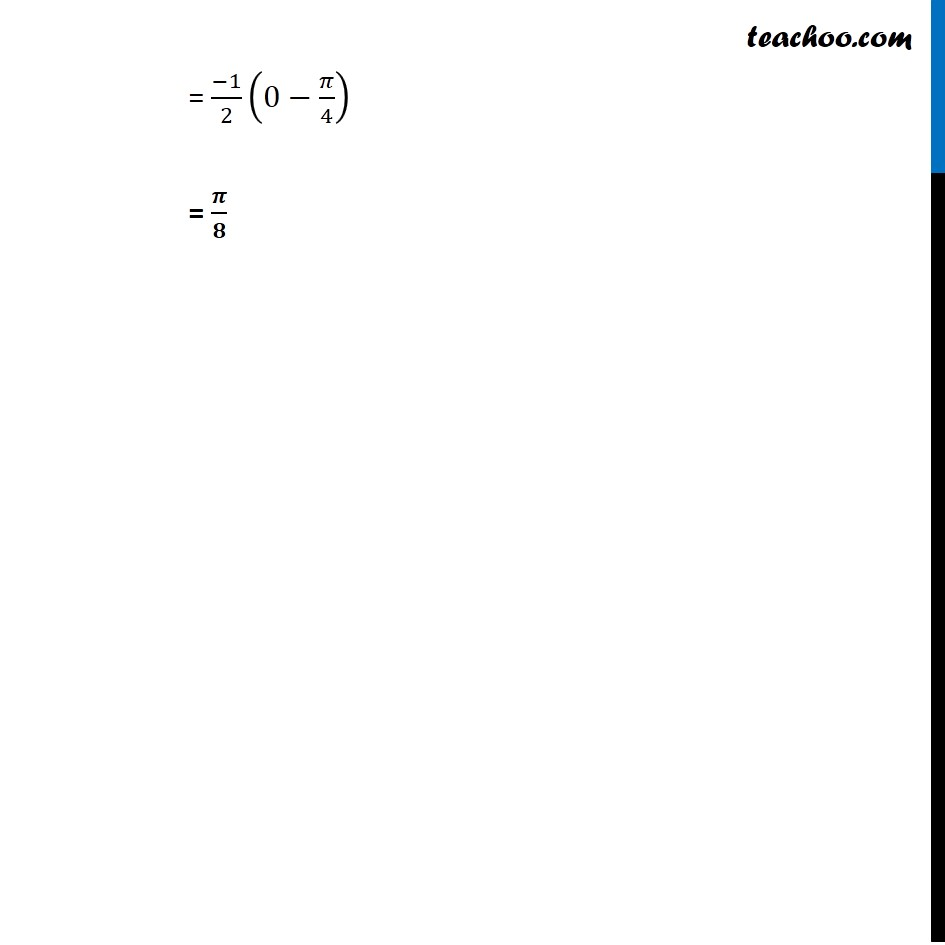Miscellaneous

Chapter 7 Class 12 Integrals
Serial order wiseLearn in your speed, with individual attention - Teachoo Maths 1-on-1 Class

### Transcript

Misc 25 Evaluate the definite integral ∫_0^(𝜋/4)▒〖(sin⁡𝑥 cos⁡𝑥)/(cos^4⁡𝑥 + sin^4⁡𝑥 ) 𝑑𝑥〗 ∫_0^(𝜋/4)▒〖(sin⁡𝑥 cos⁡𝑥)/(cos^4⁡𝑥 + sin^4⁡𝑥 ) 𝑑𝑥〗 Adding and subtracting 2 〖𝑠𝑖𝑛〗^2 𝑥 〖𝑐𝑜𝑠〗^2 𝑥 from denominator = ∫_0^(𝜋/4)▒〖(sin⁡𝑥 cos⁡𝑥)/(cos^4⁡𝑥 + sin^4⁡〖𝑥 + 2〖𝑠𝑖𝑛〗^2 𝑥 〖𝑐𝑜𝑠〗^2 𝑥 − 2〖𝑠𝑖𝑛〗^2 𝑥 〖𝑐𝑜𝑠〗^2 𝑥〗 ) 𝑑𝑥〗 = ∫_0^(𝜋/4)▒〖(sin⁡𝑥 cos⁡𝑥)/(〖〖(cos〗^2⁡𝑥 + sin^2⁡𝑥)〗^2 − 2〖𝑠𝑖𝑛〗^2 𝑥 〖𝑐𝑜𝑠〗^2 𝑥) 𝑑𝑥〗 = ∫_0^(𝜋/4)▒〖(sin⁡𝑥 cos⁡𝑥)/(1 − (4 〖𝑠𝑖𝑛〗^2 𝑥 〖𝑐𝑜𝑠〗^2 𝑥)/2) 𝑑𝑥〗 = ∫_0^(𝜋/4)▒〖(sin⁡𝑥 cos⁡𝑥)/(1 − 1/2 (2 sin⁡𝑥 cos⁡𝑥 )^2 ) 𝑑𝑥〗 = ∫_0^(𝜋/4)▒〖(sin⁡𝑥 cos⁡𝑥)/(1 − (〖𝑠𝑖𝑛〗^2 2𝑥)/2) 𝑑𝑥〗 = ∫_0^(𝜋/4)▒〖(2 sin⁡𝑥 cos⁡𝑥)/(2 − 〖𝑠𝑖𝑛〗^(2 ) 2𝑥) 𝑑𝑥〗 = ∫_0^(𝜋/4)▒〖sin⁡2𝑥/(1 + (1 − 〖𝑠𝑖𝑛〗^(2 ) 2𝑥)) 𝑑𝑥〗 = ∫_0^(𝜋/4)▒〖sin⁡2𝑥/(1 + 〖𝑐𝑜𝑠〗^2 2𝑥) 〗 𝑑𝑥 (〖𝑐𝑜𝑠〗^2 𝑥+〖𝑠𝑖𝑛〗^2 𝑥=1" " ) ("2 sin " 𝑥 cos⁡𝑥=sin⁡2𝑥 " " ) ("2 sin " 𝑥 cos⁡𝑥=sin⁡2𝑥 " " ) Let t = cos 2𝑥 𝑑𝑡/𝑑𝑥=−2 sin⁡2𝑥 (−𝑑𝑡)/2=sin⁡2𝑥 𝑑𝑥 So, our equation becomes ∫_0^(𝜋/4)▒〖sin⁡2𝑥/(1 + 〖𝑐𝑜𝑠〗^2 2𝑥) 〗 𝑑𝑥 = (−1)/2 ∫1_1^0▒𝑑𝑡/(1 + 𝑡^2 ) = (−1)/2 [〖𝑡𝑎𝑛〗^(−1) (𝑡)]_1^0 = (−1)/2 [〖𝑡𝑎𝑛〗^(−1) (0)−〖𝑡𝑎𝑛〗^(−1) (1)] = (−1)/2 (0−𝜋/4) = 𝝅/𝟖